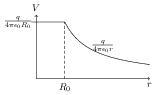# Electric Field due to Uniformly Charged Thin Spherical Shell

## Problems from IIT JEE

Problem (IIT JEE 2006):For spherical symmetrical charge distribution, variation of electric potential, $V$, with distance from centre, $r$, is given in figure. Which of the following option(s) is (are) correct,

1. Total charge within $2R_0$ is $q$.
2. Total electrostatic energy for $r\leq R_0$ is zero.
3. At $r=R_0$ electric field is discontinuous.
4. There will be no charge anywhere except at $r=R_0$.

Solution: Use relation, $E=-\frac{\mathrm{d}V}{\mathrm{d}r}$, to get electric field for given potential, \begin{align} &V(r)= \begin{cases} \frac{q}{4\pi\epsilon_0 R_0} & \text{if $r\leq R_0$,} \\ \frac{q}{4\pi\epsilon_0 r} & \text{if $r>R_0$}, \\ \end{cases}\quad; && E(r)= \begin{cases} 0 & \text{if $r\leq R_0$,} \\ \frac{q}{4\pi\epsilon_0 r^2} & \text{if $r>R_0$.} \\ \end{cases} \nonumber \end{align} Consider a spherical shell of radius $2R_0$ as Gaussian surface. Using Gauss's law for this surface, we get, \begin{alignat}{2} &q_\text{enc}=\epsilon_0\phi=\epsilon_0\cdot \frac{q}{16\pi\epsilon_0 R_0^2} \cdot 16\pi R_0^2=q.\nonumber \end{alignat} The electrostatic energy density for $r\leq R_0$ is $\frac{1}{2}\epsilon_0 E^2=0$ (since $E=0$) and hence total electric energy stored in this region is zero. The electric field is discontinuous at $r=R_0$. This can be seen by taking left and right limits, \begin{align} &\lim_{r\to R_0^-}E(r)=0, &&\lim_{r\to R_0^+}E(r)=\frac{q}{4\pi \epsilon_0 R_0^2}. \nonumber \end{align} Since electric field is continuous at all points except at $r=R_0$, there is no charge distribution except at $r=R_0$. The readers are encouraged to realize that given potential is same as the potential due to a spherical shell of radius $R_0$ having a charge $q$.